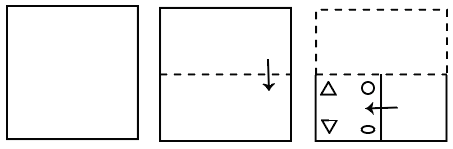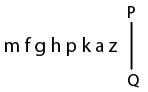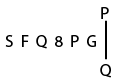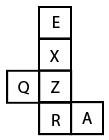# Delhi SI CPO Nov 24 Shift-1

Instructions

For the following questions answer them individually

Question 1

# Eight friends A, B, C, D, E, F, G and are sitting in a straight line, all facing the North. F is sitting between D and G. B is sitting between H and A.E is third to the left of G, who is sitting at one of the comers. H is third to the left of C. Who is sitting between A and E?Question 2

# A paper is folded and cut as shown below. How will it appear when unfolded?Question 3

# Select the correct mirror image of the given combination when the mirror is placed at 'PQ' as shown below.Question 4

# ‘A#B’ means ‘A is the husband of B’.‘A @ B’ means ‘A is the sister of B’.‘A & B’ means ‘A is the mother of B’.‘A % B’ means ‘A is the son of B’.IfS#Z@R%K#I&T#N,then which of the following statements is NOT correct?Question 5

# Select the correct mirror image of the given combination when the mirroris placed at 'PQ' as shown below.Question 6

# If the minute hand of a clock points towards the South at 12:00 a.m., then in which direction will the minute hand point at 3:00 a.m. in the same clock?Question 7

# If a denotes ‘addition’ , ‘B’ denotes ‘multiplication’, ‘C’ denotes ‘subtraction’, and ‘D’ denotes ‘division’, then what will be the value of the following expression?50D(2B5)A3 B(ISA12)C2B(18C 15) =?Question 8

# When a number is added to its next number and another number that is four times its next number, the sum of these numbers is 95. Find the number.Question 9

# Three of the following four letter-clusters are alike in a certain way and one is different. Pick the odd one out.Question 10

# A cube is made by folding the given sheet. In the cube so formed, which of the following pairs of letters will be on opposite sides?OR